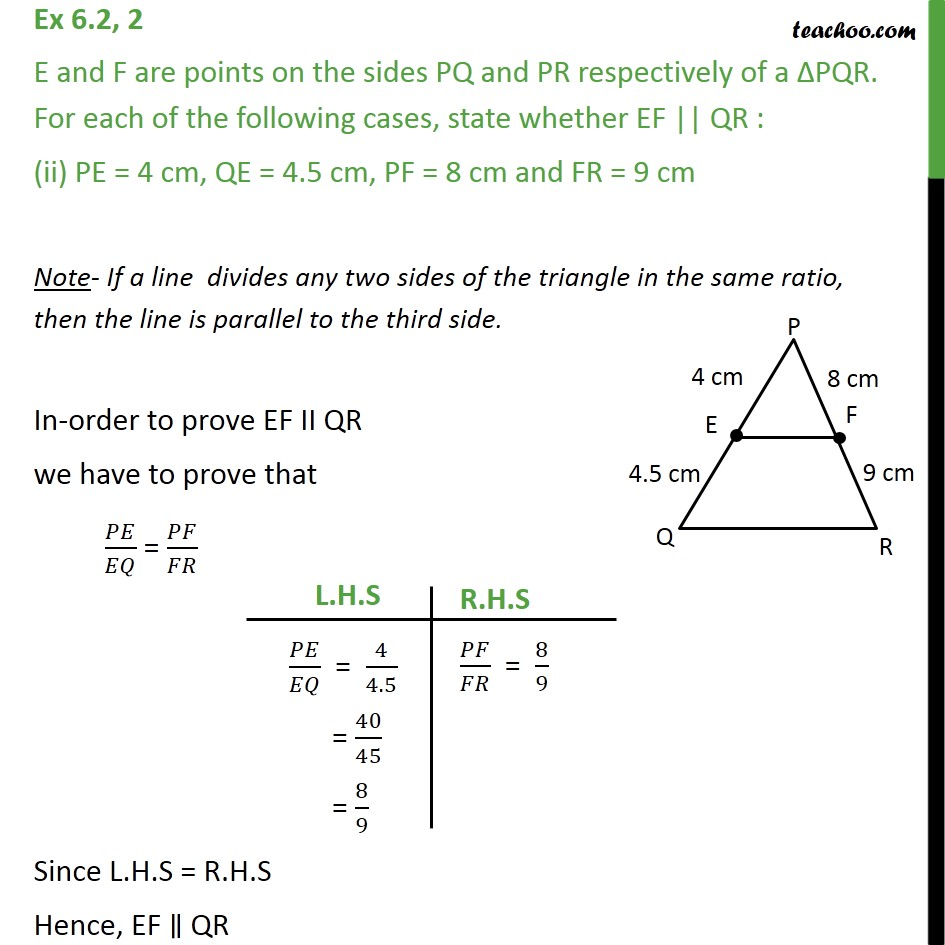Ex 6.2

Chapter 6 Class 10 Triangles
Serial order wiseLearn in your speed, with individual attention - Teachoo Maths 1-on-1 Class

### Transcript

Ex 6.2, 2 E and F are points on the sides PQ and PR respectively of a PQR. For each of the following cases, state whether EF || QR : (ii) PE = 4 cm, QE = 4.5 cm, PF = 8 cm and FR = 9 cm Note- If a line divides any two sides of the triangle in the same ratio, then the line is parallel to the third side. In-order to prove EF II QR we have to prove that / = / Since L.H.S = R.H.S Hence, EF QR